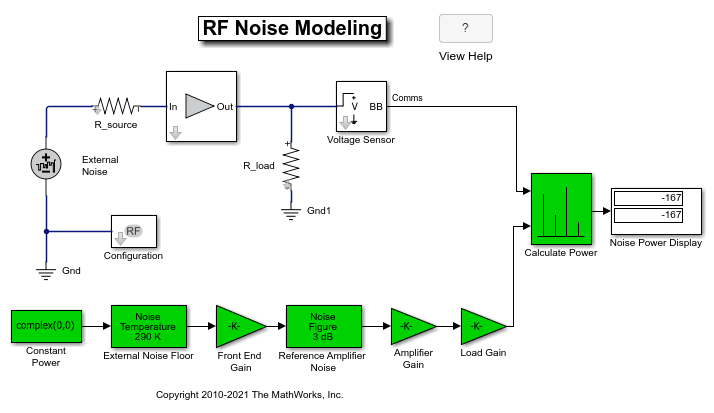Main Content

# RF Noise Modeling

This example shows how to use the RF Blockset™ Circuit Envelope library to simulate noise and calculate noise power. Results are compared against theoretical calculations and a Communications Toolbox™ reference model.

The model is shown below.### System Architecture

The RF system, shown in white, consists of:

• A Configuration block, which sets global simulation parameters for the RF Blockset system. With the Simulate Noise option checked, noise is included in the simulation.

• An External Noise source with a power spectral density ofapplied at the input. In this equation,is the Boltzmann constant,is the temperature of the source, andis the noise reference impedance. The calculated noise level of -174 dBm/Hz is used in this example. The External Noise source is an explicit signal.

• An Amplifier block with a specified power gain and noise figure.

• A Voltage Sensor (i.e. Outport) block, with the Source type parameter set to Voltage.

• Source and load resistors.

The Communications Toolbox reference system, shown in green, consists of:

• Gain blocks that model amplifier gain and loading effects.

• Two Receiver Thermal Noise blocks that model the external noise and the amplifier noise, respectively.

The Calculate Power block computes RMS noise power. Note that the Communications Toolbox signal is referenced to 1 ohm, while RF Blockset power is computed for the actual load.

The example model defines variables for block parameters using a callback function. To access model callbacks, select MODELING > Model Settings > Model Properties and click the Callbacks tab in the Model Properties window.

### Running the Example

1. Type open_system('RFNoiseExample') at the Command Window prompt.

2. Select Simulation > Run.

The Noise Power Display block verifies that the RF Blockset and Communications Toolbox noise models are equivalent.

### Computing RF System Noise

To enable noise in the RF Blockset circuit envelope environment:

• In the Configuration block dialog, select Simulate noise.

• Specify a Temperature. RF Blockset uses this value to calculate the equivalent noise temperature inside the amplifier.

• Specify the Noise figure (dB) parameter of any amplifiers or mixers in the system.

In the example, for a specified LNA gain of 4 dB and noise figure of 3 dB, the output noise is calculated using the following equations:The next equation converts the noise factor to an equivalent noise temperature.is the Temperature parameter of the RF Blockset Configuration block.The final equation calculates the output noise power.is the temperature of the SimRF™ External Noise block and the Communications Toolbox External Noise Floor block.The available noise power is the power that can be supplied by a resistive source when it is feeding a noiseless resistive load equal to the source resistance. The green External Noise Floor block generates an available power referenced to 50 ohms.

The Front End Gain block models the voltage divider due to the source resistance and the input impedance of the amplifier.

The green Reference Amplifier Noise and Amplifier Gain blocks model the noise added by the amplifier and the amplifier gain, respectively.

The output of the Communications Toolbox Amplifier Gain block is equal to the voltage across the RF Blockset R_load block.

## Support

#### Model RF Power Amplifiers and Increase Transmitter Linearity with DPD Using MATLAB

Download white paper